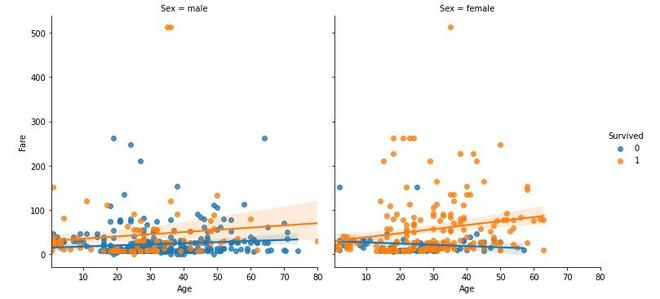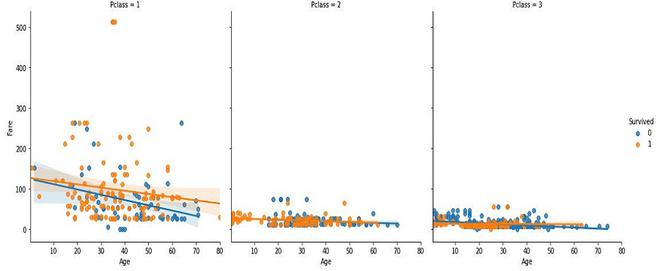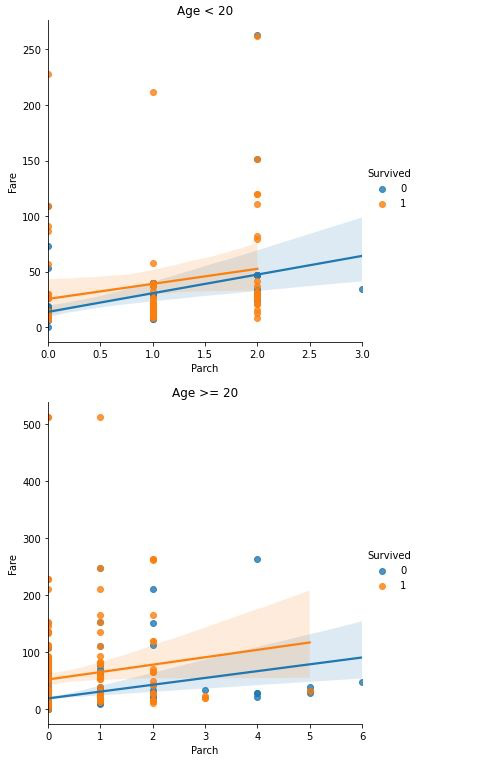Related Articles

# Conditioning Plot

• Last Updated : 05 Jul, 2021

A conditioning plot or co-plot or subset plot is a scatter plot of two variables when conditioned on a third variable. The third variable is called the conditioning variable. This variable can have both values either continuous or categorical. In the continuous variable, we created subsets by dividing them into a smaller range of values. In categorical variables, the subsets are created based on different categories.

Let’s take three variables X, Y and Z. Z be the variable which we divided into the k groups. Here, there are many ways in which a group can be formed such as:

• By dividing the data into equal size of k groups.
• By dividing the data into different clusters on the basis of scatter plot.
• By dividing the range of data points into equal values.
• The categorical data have natural grouping on the basis of different categories of the dataframe.

Then, we plot n rows and m columns matrix where n*m >= k. Each set of (row, column) represents an individual scatter plot, in which each scatters plot consists of the following components.

• Vertical Axis: Variable Y
• Horizontal Axis: Variable X

where, points in the group corresponding to row i and column j are used.

The conditioning plot provides the answer to the following questions:

• Is there any relationship between the two variables?
• If there is a relationship then, does the nature of the relationship depend upon the third variable?
• Do different groups in the data behave similarly?
• Are there any outliers in the data?

## Python3

 `# code``%` `matplotlib inline``import` `numpy as np``import` `seaborn as sns``import` `matplotlib.pyplot as plt``import` `pandas as pd` `# load training file for titanic dataset``titanic_dataset ``=``pd.read_csv(``'train.csv'``)` `# head of dataset``titanic_dataset.head()` `# conditioning plot on the basis of categorical variables``sns.lmplot(x``=``'Age'``, y ``=``'Fare'``,hue``=``'Survived'``, col ``=``'Sex'``,data``=``titanic_dataset)``sns.lmplot(x``=``'Age'``, y ``=``'Fare'``,hue``=``'Survived'``, col ``=``'Pclass'``,data``=``titanic_dataset)` `# conditioning plot on the basis of continous variables``df1, df2 ``=` `titanic_dataset.loc[titanic_dataset[``'Age'``] < ``20` `] ,``    ``titanic_dataset.loc[titanic_dataset[``'Age'``] >``=` `20` `]`  `lm ``=` `sns.lmplot(x``=``'Parch'``, y ``=``'Fare'``,hue``=``'Survived'``,data``=``df1)``ax1 ``=``lm.axes``ax1``=``plt.gca()``ax1.set_title(``'Age < 20'``)` `lm_2 ``=` `sns.lmplot(x``=``'Parch'``, y ``=``'Fare'``,hue``=``'Survived'``,data``=``df2)` `ax2 ``=``lm_2.axes``ax2``=``plt.gca()``ax2.set_title(``'Age >= 20'``)`Conditional Plot on the basis of SexConditional Plot on the basis of Passenger_ClassConditional Plot on the basis of Age

### References:

Attention reader! Don’t stop learning now. Get hold of all the important Machine Learning Concepts with the Machine Learning Foundation Course at a student-friendly price and become industry ready.

My Personal Notes arrow_drop_up